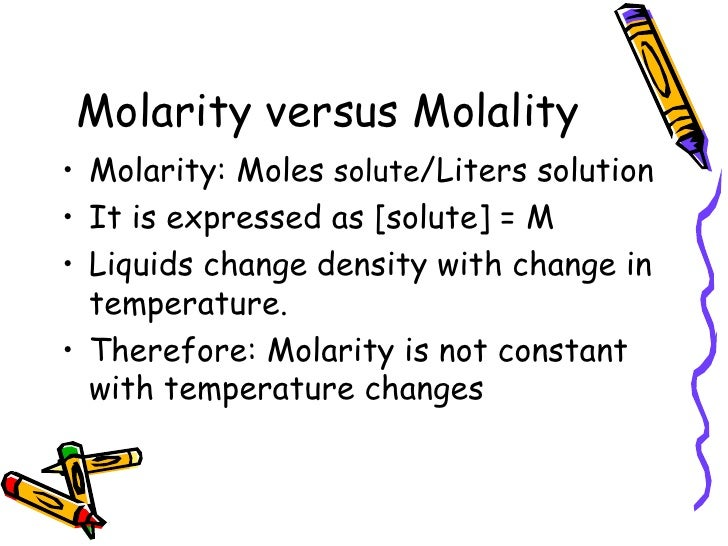# What concentration measure changes with temperature?

Jan 27, 2014

Molarity changes with temperature.

#### Explanation:

Molarity changes with temperature.

Molarity is moles of solute per litre of solution. Water expands as the temperature increases, so the volume of the solution also increases. You have the same number of moles in more litres, so the molarity is less at higher temperatures.

EXAMPLE

Assume that you have a solution that contains 0.2500 mol of NaOH in 1.000 L of solution (0.2500 M NaOH) at 10 °C. At 30 °C, the volume of the solution is 1.005 L, so the molarity at 30 °C is

$\frac{0.2500 m o l}{1.005 L}$ = 0.2488 M

This may not seem like a big difference, but it is important when you need more than two significant figures in a calculation.

MORAL: If you use molarities in your calculations, make sure that they are all measured at the same temperature.